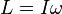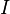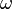# Angular momentum facts for kids

Kids Encyclopedia Facts

The angular momentum (L) of an object rotating about an axis is the product of its moment of inertia and its angular velocity:$L = I \omega$

where$I$ is the moment of inertia (resistance to angular acceleration or deceleration, equal to the product of the mass and the square of its perpendicular distance from the axis of rotation);$\omega \$ is the angular velocity.

Angular momentum is a conserved quantity—an object's angular momentum stays constant unless an external torque acts on it.

## Images for kidsAngular momentum Facts for Kids. Kiddle Encyclopedia.